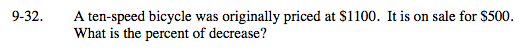### Home > MC2 > Chapter 9 > Lesson 9.1.3 > Problem9-32

9-32.

A ten-speed bicycle was originally priced at $1100. It is on sale for$500. What is the percent of decrease? Homework Help ✎The percent of decrease is the value of the decrease as a percent of the original price.

Decrease: $1100 -$500 = $600$600 = (x)(\$1100)

55%